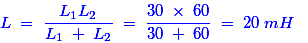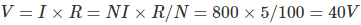# MCQs on Electromagnetic Fields

##### Page 17 of 20. Go to page 1 2 3 4 5 6 7 8 9 10 11 12 13 14 15 16 17 18 19 20
01․ A pure inductor when connected across 220 V, 50 Hz supply consumes 100 W. From the above statement it can be concluded that
the inductor is made of thin wire.
the inductor has ferrite core.
the inductor is non linear.
the above statement is false.

The pure inductor does not consume any actual power from source. Hence, the above statement is false.

02․ Which of following is true for a purely inductive circuit ?
Any capacitance even if present in the circuit will not be charged.
Reactive power of the circuit is zero.
Actual power of the circuit is zero.
Apparent power of the circuit is zero.

A pure inductor has 90° power angle between voltage and current. Hence, cos90° is zero and thereby the actual power P = VIcos90° is also equal to zero.

03․ The main purpose of choke is
to block low frequencies.
to pass high frequencies.
to pass low frequencies.
none of above.

The choke is nothing but one type of inductor. The main purpose of it is to block high frequencies and pass low frequencies.

04․ RF inductor are characterized by
high current rating and high resistance.
low current rating and low resistance.
high current rating and low resistance.
low current rating and high resistance.

Because the RF inductor suffers from the proximity effects and that leads to do some malfunction in it. That is why we use to characterize them by low current rating and high resistance to protect the RF inductor from proximity effects.

05․ A crack in the magnetic path of an inductor will result in
unchanged inductance.
increased inductance.
zero inductance.
reduced inductance

A crack in the magnetic path of an inductor leads to collapse in the strength of magnetic field. If it looses the strength of its magnetic field, then obviously there could be a chance of reduction in its inductance.

06․ An open coil has
zero resistance and inductance.
infinite resistance and zero inductance.
infinite resistance and normal inductance.
zero resistance and high inductance.

An open coil is nothing but the ideal inductor. The ideal inductor has zero resistance in nature that allows more currents to flow through it. Hence, it creates high magnetic field and thereby the inductance is also high.

07․ inductance (L2) in parallel without mutual coupling will be
5 mH.
15 mH.
20 mH.
10 mH.

For parallel connected inductor, the equivalent inductor isIf the mutual inductance between them is zero, the equivalent inductance would be L / 2 for two self-induced inductors in parallel. Hence, the total inductance is Ltotal = 20 / 2 = 10 mH.

08․ In above problem, if the inductance L1 and L2 in series aiding with 30 mH mutual inductance, then total inductance will be
50 mH.
100 mH.
150 mH.
200 mH.

For series connected inductor, the equivalent inductor is Lseries = L1 + L2. So, Lseries = 30 + 60 = 90 mH. If this combination is connected with one more 30 mH mutual inductance, then the equivalent inductor Leq = Lseries + (Lmutual × 2) = 90 + (2 × 30) = 150 mH.

09․ The force experienced by a current carrying conductor lying parallel to a magnetic field is
BIL.
zero.
Bil sinθ.
Bil cosθ.

Force experienced by a current carrying conductor = B.I.L.Sinθ. If the conductor is parallel to the magnetic field, then θ = 0. So, Sinθ = 0.

10․ A magnetic circuit requires 800 AT to produce a certain quantity of flux in a magnetic circuit. If its excitation coil has 100 turns and 5 Ohm resistance, the voltage to be applied in exciting coil is
40 V.
60 V.
80 V.
8 V.<<<1516171819>>>